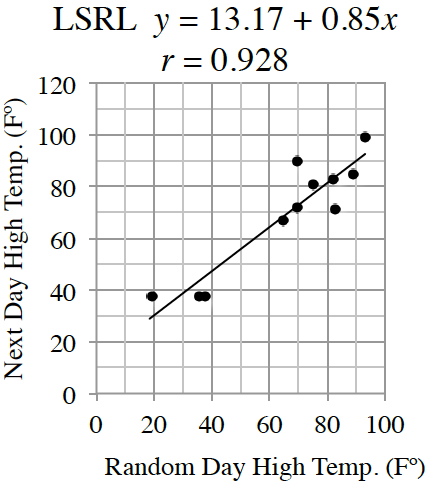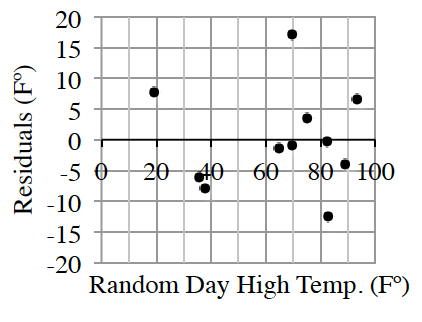### Home > GB8I > Chapter 7 Unit 8 > Lesson INT1: 7.1.7 > Problem7-83

7-83.

Mitchell likes to study the weather. He is fascinated by the sophistication of the computer models used to make weather predictions. Mitchell wonders if he can make his own model to predict the next day’s high temperature in his area based only on today’s high temperature. He selects $11$ days at random and gets the temperatures from the Internet. The results from his computer spreadsheet are shown.Random Day ($F^◦$)

Next Day ($F^◦$)

$93.2$

$99$

$69.8$

$71.6$

$82.9$

$71.1$

$82.4$

$82.9$

$19.4$

$37.4$

$69.8$

$89.6$

$35.6$

$37.4$

$89.1$

$84.9$

$37.9$

$37.4$

$75.2$

$80.6$

$64.9$

$66.9$

1. Write a few sentences that describe the association. Remember to include interpretations of slope and $R^{2}$.

Read Math Note box in section 4.2.4 on correlation coefficients and the meaning of R².
Also remember to look at the axis labels when working with slope.
Be sure to discuss outliers as well.

2. Use the graph to estimate the largest residual. To what point does it belong?

The largest residual belongs to the day after the $69.8$ degree day with a value of about $17$ degrees.

3. Using the $LSRL$ model, estimate tomorrow’s high temperature based on today’s high temperature of $55º$ in Mitchell’s area. Use appropriate precision.

Let $x = 55$ in the given $LSRL$ equation.

$60$ degrees because $13.17 + .85(55) ≈ 60.0º$.

4. Consider the upper and lower bounds of the prediction Mitchell made in part (c) above. Is Mitchell’s model ready to replace the complex models of the professional meteorologists? Support your answer.

Draw upper and lower boundary lines on the $LSRL$ graph. How far apart are these lines?